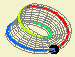## Paths on a surface### Moebius band - fundamental group[see app]

In the Moebius strip the study of homotopies of paths is not as simple as in the plane. Notice, for instance, the closed path that goes around the strip. As we cannot move the base, it is not possible to deform continuously this closed path to a point; hence, these two paths (the closed path and the base point) are not homotopic. This idea can be explored intuitively in the app.

Considering this example and after experimenting with the app, what can we conjecture about homotopies of paths in the Moebius strip?

One important observation is that it is related to the number of times a path goes around the strip. In fact, it can be shown this determines each homotopy class. Given two paths, there is an homotopy if and only if the number of times each goes around the strip is the same (computed after closing the paths connecting the end points by a shortest path, if necessary).

Hence, in the Moebius strip, each homotopy class of closed paths is characterized by one integer. This determines a correspondence between the set of homotopy classes and the integers - the fundamental group of the Moebius strip is isomorphic to $$\mathbb{Z}$$.

...

...

This correspondence can also be understood by the lift of the Moebius strip over the plane. Considering a base point in the Moebius strip and a lift of the strip over a planar strip, each path in the Moebius strip admits a single lift to a path in the planar strip, with respect to a choice of a start point the planar strip. Therefore, the equivalence class represented by the closed path in the Moebius strip is determined by the end point of the corresponding path in the planar strip; this point can be characterized by an integer (with respect to its position to the left or right of the start point).